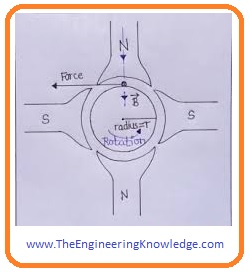Hello, fellows, I hope all of you are enjoying your life. In today’s tutorial, we will have a look at the Internal Generated Voltage and Torque Equation of DC Machines. Like AC machines there are 2 types of DC machines first one is DC motor and the second one is DC generator. There are some important factors in dc machines like commutation in which AC is converted into DC. There are some problem comes during commutation like armature reaction and L di/dt voltage. All these factors play an important role in the operation of these dc machines.

If the load is connected with the dc machine armature current starts to flow due to that flux produced in armature windings. The flux of poles at stators linked with the armature winding flux and force acts on a rotor of the machine so it rotates. Due to this force-torque applied on the machine that rotates machine. In today’s post, we will have a look at how this torque produced in the dc machine and find its equation also calculate the internal generated voltage in the machines. So let’s get started with the Internal Generated Voltage and Torque Equation of DC Machines.

#### Internal Generated Voltage and Torque Equation of DC MachinesVoltage Induced in DC Machines

• There are 3 main factors over which dc machines voltages depend on.
• Flux ø of the machine.
• A rotation speed of the machine.
• Third, is constant over which construction of dc machine rely.
• The value of voltage at the armature winding of DC machine is no of a winding conductor in a single current path with the multiple of voltage in every conductor.
• The voltage induced in a single conductor under effect of pole is given as.

eind = e = vBI

• The value of voltage at the armature of a machine is given as.

EA = ZvBI/a——(1)

• In this equation, ‘Z’ is no of a conductor in armature winding and ‘a’ is current paths.
• The rotation speed of every conductor is given as (V=rw) in this equation ‘r’ is radius of rotor.
• So equation (1) becomes.

EA = ZrwBI/a

• This equation can be written in more comprehensive way as we know flux of poles is equal to the product flux density and area of poles.

ø= BAp

• the area of rotor is like the cylinder son written as.

A= 2πrl

• If there are P poles on the machine, then the portion of the area associated with each pole is the total area A divided by the number of poles P.
• If the no of poles in a machine is ‘P’ so the area cover by all poles will be equal to the.

AP= A/P= 2πrl/P

• So total flux in a machine will be.

ø= BAp = B(2πrl/P)

ø =2πrl B /P

• so internal generated voltage in dc machine will become.

EA = ZrwBI/a

EA =(ZP/2πa)( ZπrBI/P)w

EA =(ZP/2πa)(øw)

EA =K(øw)

Here K =(ZP/2πa)

• In industries, the rotation speed of machines is measured in (rpm) instead of radians per second. So we have.

W=(2π/60)n

• So induced voltage equation in terms of rpm can be written as.

EA =K’øn

K’=(ZP/60a)

#### Torque in DC Machines• The induced torque in dc machines depends on three main factors that are listed here.
• Flux ø in a machine.
• Armature current (IA) in the machine.
• The third is constant that depends on structure of machine.
• The torque induced on armature windings is equal to the product of no of a conductor in armature winding and torque in every conductor.
• The value of torque on every conductor of armature winding is given as.

Tcond=rIconcLB

• If the no of current paths in a machine is ‘a’ so the current IA will divide into each current paths.
• So current in a conductor of armature winding is given as.

Icond= IA/a

• The torque in a single conductor will be given as.

tcond= (rIALB)/a

• as the no of a conductor are ‘Z’ thus the total induced torque in the armature winding of rotor is given as.

Tind=(ZrIALB)/a

• The flux of each pole of machine is given as.

Ø=BAP=B(2πrL)/P

= B2πrL/P

• So total induced torque can be written as.

Tind =(ZP/2πa)(ØIA)

Tind=K ØIA

K =ZP/2πa

• Both voltage and torque equations that we find are just estimations since not all conductors of armature windings are under the effect of poles flux.
•  To calculate the accurate value of internal generated voltage and torque induced use conductor that is under the effect of poles not all conductors of armature windings.

So friends that is the detailed post on the Internal Generated Voltage and Torque Equation of DC Machines if you have any query ask in comments. Thanks for reading see you in next post.